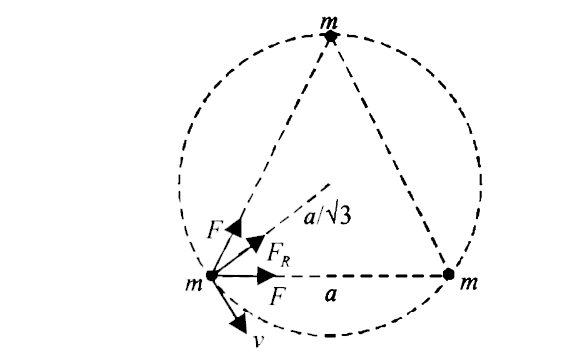# Three particles, each of mass m, are situated at the vertices of equilateral triangle of side length a. The only forces. It is desired that each parti

31 views
in Physics
closed
Three particles, each of mass m, are situated at the vertices of equilateral triangle of side length a. The only forces. It is desired that each particle moves in a circle while maintaining the original mutual speration a. Find the intial velocity that should be given to each particle and also the time period of the circular motion.

by (75.0k points)
selected

The radius of the circle r = (2)/(3) sqrt(a^2 - (a^2)/(4)) = (alpha)/(sqrt3)
Let v be the velocity given . The centripetal force is provied by the resultant gravitational attraction of the two masses.
F_F = sqrt(F^2 +F^2 +2F^2 cos 60^@)
=sqrt3 F =sqrt3G (mxxm)/(a^2)
:. sqrt3G (m^2)/(a^2) = (mv^2)/(r )
((mv^2)/(r ) = centripetal force)
v^2 = (sqrt3Gmr)/(a^2) = sqrt(3Gma)/(a^2xxsqrt3) rArr v = sqrt((Gm)/(a))
Time period of circular motion
T = (2pir)/(v) = (2pia//sqrt3)/(sqrt(Gm)/(a)) = 2pisqrt((a^3)/(3Gm))